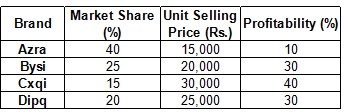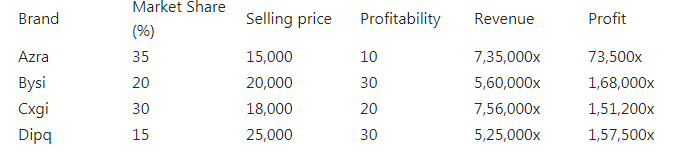# There are only four brands of entry | CAT DILR Questions | Data Interpretation | Table

## CAT 2018 – Slot 2 - Data Interpretation - There are only four brands of entry

##### There are only four brands of entry level smartphones called Azra, Bysi, Cxqi, and Dipq in a country. Details about their market share, unit selling price, and profitability (defined as the profit as a percentage of the revenue) for the year 2016 are given in the table below:In 2017, sales volume of entry level smartphones grew by 40% as compared to that in 2016. Cxqi offered a 40% discount on its unit selling price in 2017, which resulted in a 15% increase in its market share. Each of the other three brands lost 5% market share. However, the profitability of Cxqi came down to half of its value in 2016. The unit selling prices of the other three brands and their profitability values remained the same in 2017 as they were in 2016.

Q. 1: The brand that had the highest revenue in 2016 is:
1. Dipq
2. Bysi
3. Cxqi
4. Azra

##### Q. 2: The brand that had the highest profit in 2016 is:1. Azra2. Bysi3. Cxqi4. Dipq

Q. 3: The brand that had the highest profit in 2017 is:
1. Dipq
2. Bysi
3. Cxqi
4. Azra

Q. 4:The complete list of brands whose profits went up in 2017 from 2016 is:
1. Azra, Bysi, Cxqi
2. Bysi, Cxqi, Dipq
3. Cxqi, Azra, Dipq
4. Azra, Bysi, Dipq

Question 1 and 2

Let the total market size in 2016 be 100x units. The sales of Azra, Bysi, Cxqi and dipq would be 40x,25x,15x and 20x units respectively.

So the revenue of Azra = 40x × 15,000 = 6,00,000x ,

profit = 10% of 6,00,000x = 60,000x

Revenue of Bysi = 25x ×20,000 = 5,00,000x

Profit = 30% of 500000x = 1,50,000x

Revenue of Cxgi = 15x × 30,000 = 4,50,000x

Profit = 40 % of 4,50,000x = 1,80,000x

Revenue of Dipq = 20x × 25,000 = 5,00,000x

Profit = 30 % of 5,00,000x = 1,50,000x

1. the for the year brand with the highest revenue is Azra.

2. maximum profit is 1,80,000 for Cxgi.

Question 3 and 4

In 2017 Sales volume increased by 40% as compare to sales volume in 2016 so new sales volume = 140x and similarly market share of Cxgi went up by 15% point and others get down by 5 percentage point. The information can be tabulated as given bellow (Profit and revenue can be calculated as previous question )

Total Sales volume = 140x3. the profit is the highest for Bysi .

4. The profits increased for Azra (60,000 to 73,500) for Bysi (1,50,000 to 1, 68,000) and Dipq (1,50,000 to 1,57,500)

### Past Year Question Paper & SolutionsCounselling Session
By IIM Mentor

### Free Material Area